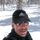cancel
Showing results for
Did you mean:Helper I

## Creating a measure that calls another measure......

Hello,

I am hoping you can help me.  I have a table with a ton of data and I'm trying to create one measure that calculates what I need correctly.

So, I have a column named "Key", and based on the value of "Key", I need my other column "Results" to perform the proper math.  I have 5 measures created with the proper formulas, however I don't know how to call them.

My key is either "PAPER-FT", "PAPER-LB", "FILM-FT", "FILM-LB", or "FOAM-LB", and the measures I have created are called the identical name so it stays easy (so I thought).  So if Key = "PAPER-FT" I want it to run the calculation in my measure "PAPER-FT", etc.

I konw how to do it with a calculated column, and it works, however it's so big that if I try to add anymore columns my BI crashes and says it is out of memory.  I know that's b/c it has to do the math for all those calculations then assign them to each row.  That is why I am trying to do this with a measure, but I'm stumped.

I hope I explained this clear enough, please let me know if you have any questions, and thank you very much for your help with this!

1 ACCEPTED SOLUTIONSuper User

Hi @DCPics

Try this: Dummied up two measures and called them from the third.
Let me know if you have any questions.

If this solves your issues, please mark it as the solution, so that others can find it easily. Kudos 👍are nice too.
Nathaniel

``Paper-FT calc = 1+2``

``Paper-LB calc = 1-2``

``Results = IF(MAX(myTable[Type])= "Paper-FT",[Paper-FT calc],IF(max(myTable[Type])="Paper-LB",[Paper-LB calc]))​``Proud to be a Super User!

4 REPLIES 4Super User

Hi @DCPics

Try this: Dummied up two measures and called them from the third.
Let me know if you have any questions.

If this solves your issues, please mark it as the solution, so that others can find it easily. Kudos 👍are nice too.
Nathaniel

``Paper-FT calc = 1+2``

``Paper-LB calc = 1-2``

``Results = IF(MAX(myTable[Type])= "Paper-FT",[Paper-FT calc],IF(max(myTable[Type])="Paper-LB",[Paper-LB calc]))​``Proud to be a Super User!Helper I

Thank you very much for your help with this!  I really appreicate it.  I didn't realize you could do IF's in a measure.Super User

Hi @DCPics , you are welcome!
Try @MattAllington 's book, Supercharge Power BI.

Nathaniel

Proud to be a Super User!Helper I

Thanks for the tip on the book, I'm going to look it up now.  Thanks again for the help.